Courses

# Friction Force NAT Level - 1

## 10 Questions MCQ Test Basic Physics for IIT JAM | Friction Force NAT Level - 1

Description
This mock test of Friction Force NAT Level - 1 for Physics helps you for every Physics entrance exam. This contains 10 Multiple Choice Questions for Physics Friction Force NAT Level - 1 (mcq) to study with solutions a complete question bank. The solved questions answers in this Friction Force NAT Level - 1 quiz give you a good mix of easy questions and tough questions. Physics students definitely take this Friction Force NAT Level - 1 exercise for a better result in the exam. You can find other Friction Force NAT Level - 1 extra questions, long questions & short questions for Physics on EduRev as well by searching above.
*Answer can only contain numeric values
QUESTION: 1

### A block of mass 4kg is kept on ground. The coefficient of friction between the block and the ground is 0.80. An external force of magnitude 30N is applied parallel to the ground. The resultant force (in N) exerted by the ground on the block is : (g =10 m/s2)

Solution: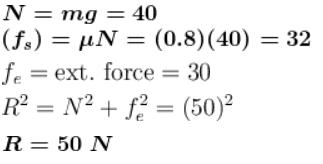*Answer can only contain numeric values
QUESTION: 2

### A block of mass 15kg is resting on a rough inclined plane as shown in figure. The block is tied up by a horizontal string which has a tension of 50N. The coefficient of friction between the surface of contact is  (g = 10 m/s2)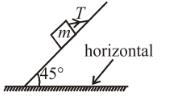Solution:

The free body diagram of the block is
N is the normal reaction exerted by inclined plane on the block.
Applying Newton’s second law to the block along the normal to the incline.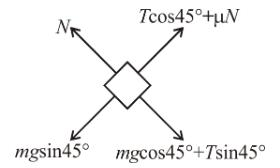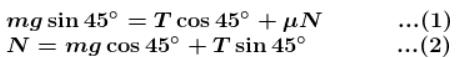Solving we get μ = 1/2

*Answer can only contain numeric values
QUESTION: 3

### An external force of 6 N is applied on body at rest at time t = 0.  At  t = 1 sec direction of force is reversed. Find the speed ms–1 of body when it comes back to the initial position.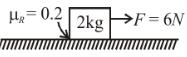Solution: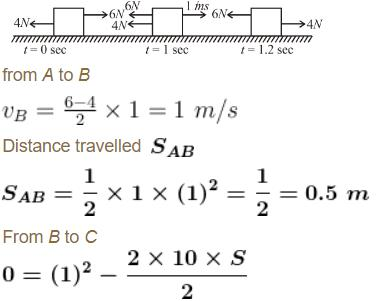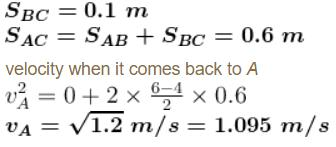*Answer can only contain numeric values
QUESTION: 4

Figure shows a block kept on a rough inclined plane. The maximum external force along the plane downwards for which the block remains at rest is 1N while the maximum external force along the incline upwards for which the block is at rest is 7N. The coefficient of static friction μ is :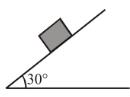Solution:

When block is about to slide down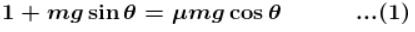When block in about to slide up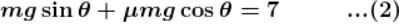From (1) and (2)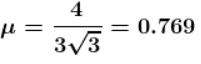*Answer can only contain numeric values
QUESTION: 5

What is the maximum value of the force F (in N) such that the block shown in the arrangement does not move?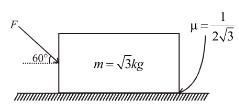Solution: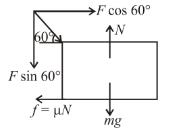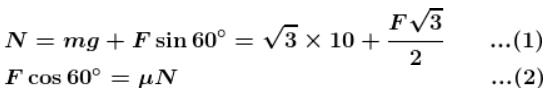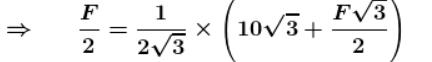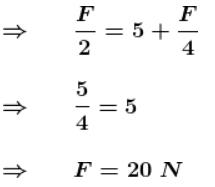*Answer can only contain numeric values
QUESTION: 6

A block is given velocity 10 m/s along the fixed inclined as shown in figure from the bottom of the inclined plane. Find the total distance traveled (in meter) along the inclined plane, if the coefficient of friction is equal to 0.5 :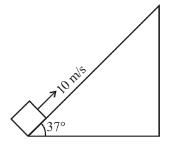Solution:

distance traveled along inclined plane till it stops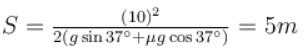But friction force is not sufficient to stop there, so it will come back to the bottom of the inclined plane.
Total distance traveled  5 + 5 = 10m

*Answer can only contain numeric values
QUESTION: 7

A uniform rope so lies on a table that part of it lays over. The rope begins to slide when the length of  hanging part is 25% of entire length. The coefficient of friction between rope and table is :

Solution: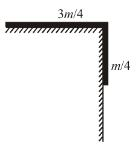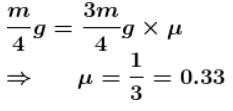*Answer can only contain numeric values
QUESTION: 8

With reference to the figure shown, if the coefficient of friction at the surface is 0.42, then the forces F (in N) required to pull out the 6.0kg block with an acceleration of 1.50 m/s2 will be :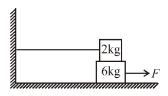Solution: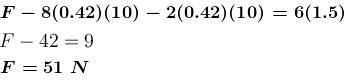*Answer can only contain numeric values
QUESTION: 9

Starting from rest a body slides down a 45° inclined plane in twice the time it takes to slide down the same distance in the absence of friction. The coefficient of friction between the body and the inclined plane is :

Solution: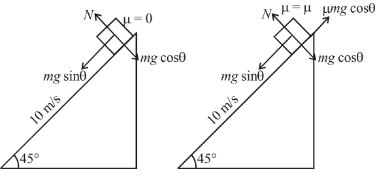Let acceleration in 1st case is a1 and that in second case is a2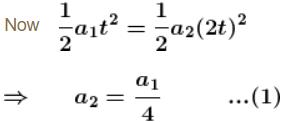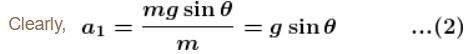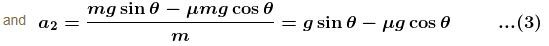From (1), (2) and (3), we get
μ = 0.75

*Answer can only contain numeric values
QUESTION: 10

A body of mass 10kg lies on a rough horizontal surface. When a horizontal force of F newtons acts on it, it gets an acceleration of 5 m/s2. When the horizontal force is doubled, it gets an acceleration of 18 m/s2. The coefficient of friction between he body and the horizontal surface is :

Solution: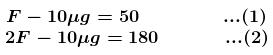From (1) and (2)
μ = 0.8